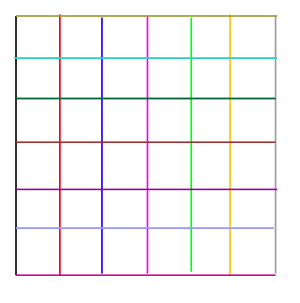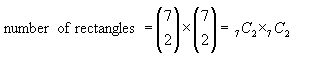SEARCH HOMEMath Central Quandaries & QueriesQuestion from Maria, a parent: Given a six by six square, how rectangles are there in the square?Hi Maria.

A rectangle will have four sides: two vertical and two horizontal.

There are 6 "spaces", so there are 5 lines between them plus a line on each edge. So there are seven possible vertical lines and seven possible horizontal lines.This means we need to find the number of ways of choosing 2 vertical lines from a group of seven and multiply that by the number of ways of choosing 2 horizontal lines from a group of seven to find the answer.

This can be written in two common forms:Can you take it from here?

Cheers,
Stephen La Rocque.Math Central is supported by the University of Regina and The Pacific Institute for the Mathematical Sciences.